Alkanes is the first functional group that is taught in Organic Chemistry in the GCE A Level H2 Chemistry syllabus. On first glance, it is a relatively easy topic with not much content as it only has one reaction which is free radical substitution. Most students would probably just memorize the free radical substitution mechanism and conclude their study for this chapter. But it is not so simple. Here is a sneak peek of the 3 commonly tested concepts in Alkanes that students will learn in our A Level Chemistry tuition programme.

1) Number and Ratio of Different Types of Products Formed

Supposed you do a free radical substitution on 2-methylbutane using Cl2,

a) how many different types of products would you get

b) would they be present in equal amount? If not, in what ratio would the products be obtained?

To answer the first question, draw out the structure of 2-methylbutane to see how many different types of hydrogen atoms you can substitute.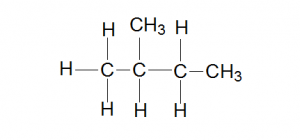Can you see how many different types of hydrogen atoms are there? If you can’t, how about converting it into a more condensed formula?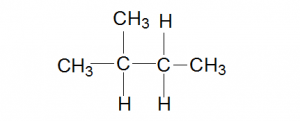There are 4 different types of hydrogen atoms that can be substituted and hence 4 different products can be obtained.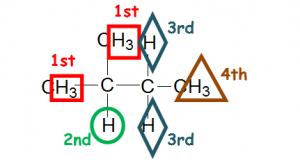Some students may feel that substituting at the 1st and 4th hydrogen will be the same, if you are not sure, just name the product based on IUPAC rules and it will give you different names if they are different products!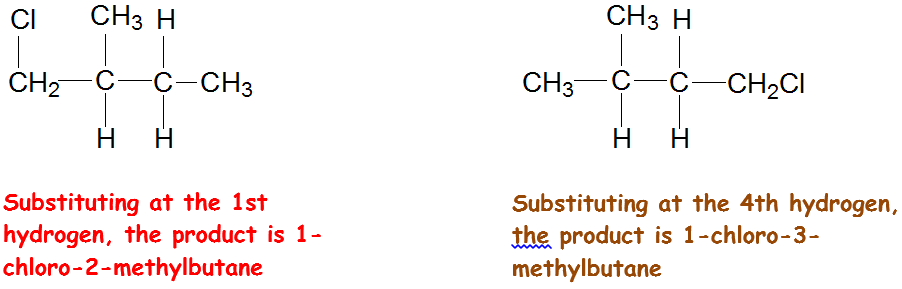Hence the 1st and the 4th hydrogen are not the same as substitution of the 1st and the 4th hydrogen gives you different products.

Coming to the 2nd question, do you think the products would be formed in equal amount? The ratio of products formed depend on the number of each type of hydrogen atoms present.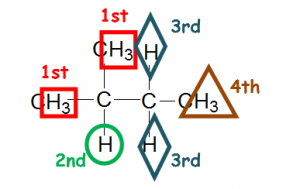Hence the products formed will be in the following ratio: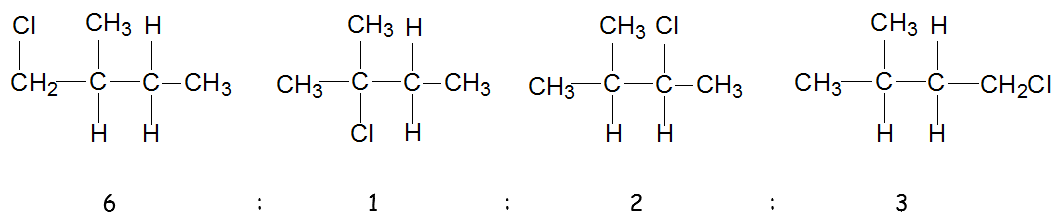This is the main factor (based on probability) used in deriving the ratio of products formed. However, another factor you need to be aware of is based on the stability of the free radicals. It will also affect the ratio of the products formed. If you consider this factor as well, then the actual ratio of the products formed will not be 6:1:2:3. How would you attempt a question which take both factors (probability and stability) into account in deriving the actual ratio? This was tested in past years internal exam but not yet at A levels.

See the 2nd & 3rd commonly tested concepts in Alkanes: Difference in Reactivity and Free Radical Mechanism.
Found these useful? Students will benefit more during class. Click on the button below to register for Ms Sim’s Chemistry tuition.Check out our A Level Maths tuition in Singapore, and A Level Physics tuition.